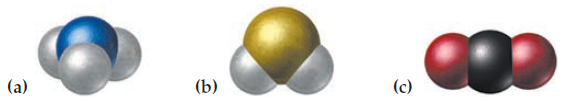×
Get Full Access to Introductory Chemistry - 5 Edition - Chapter 5 - Problem 88p
Get Full Access to Introductory Chemistry - 5 Edition - Chapter 5 - Problem 88p

×

# Write a molecular formula for each molecular model. (White = hydrogen; red = oxygen;ISBN: 9780321910295 34

## Solution for problem 88P Chapter 5

Introductory Chemistry | 5th Edition

• Textbook Solutions
• 2901 Step-by-step solutions solved by professors and subject experts
• Get 24/7 help from StudySoup virtual teaching assistantsIntroductory Chemistry | 5th Edition

4 5 1 280 Reviews
14
2
Problem 88P

Write a molecular formula for each molecular model. (White = hydrogen; red = oxygen; black = carbon; blue = nitrogen; yellow = sulfur)Step-by-Step Solution:
Step 1 of 3

Solution 88P

Write a molecular formula for each molecular model. (White = hydrogen; red = oxygen; black = carbon; blue = nitrogen; yellow = sulfur)

(a)Ans. NH3

(b)Ans. SH2

(c)Ans. CO2

Step 2 of 3

Step 3 of 3

##### ISBN: 9780321910295

Introductory Chemistry was written by and is associated to the ISBN: 9780321910295. Since the solution to 88P from 5 chapter was answered, more than 368 students have viewed the full step-by-step answer. This textbook survival guide was created for the textbook: Introductory Chemistry, edition: 5. This full solution covers the following key subjects: Molecular, nitrogen, carbon, formula, hydrogen. This expansive textbook survival guide covers 19 chapters, and 2046 solutions. The answer to “?Write a molecular formula for each molecular model. (White = hydrogen; red = oxygen; black = carbon; blue = nitrogen; yellow = sulfur)” is broken down into a number of easy to follow steps, and 23 words. The full step-by-step solution to problem: 88P from chapter: 5 was answered by , our top Chemistry solution expert on 05/06/17, 06:45PM.

Unlock Textbook Solution